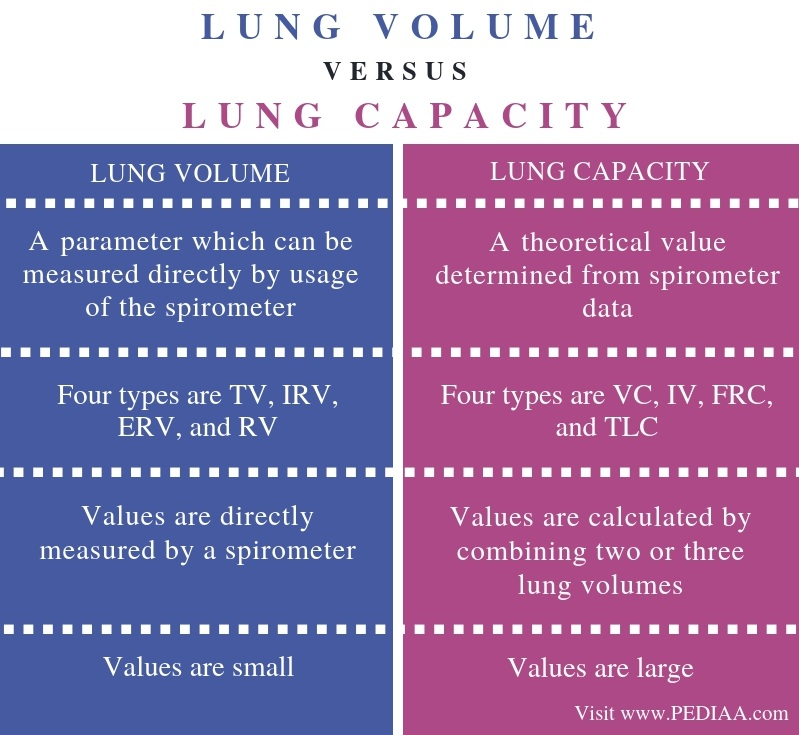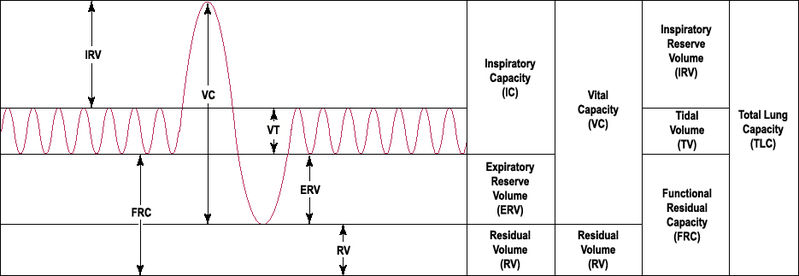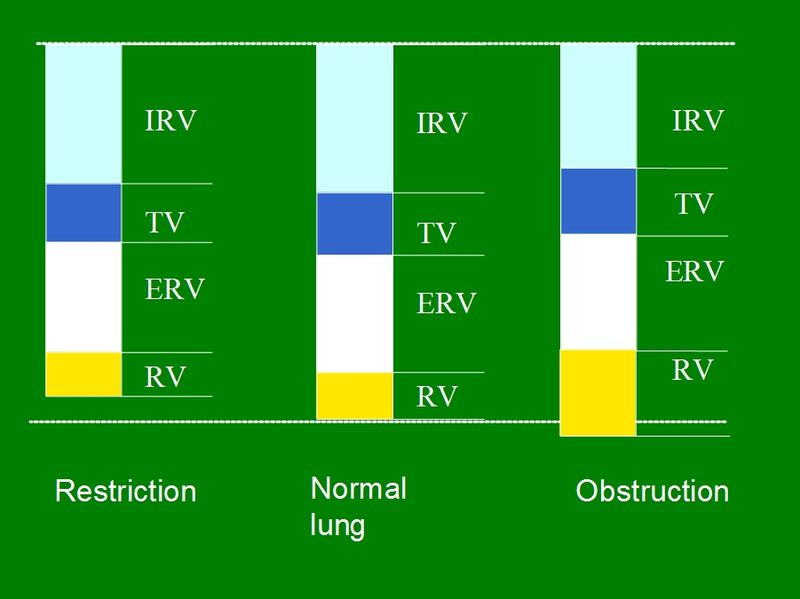What is the Difference Between Lung Volume and Lung Capacity

The main difference between lung volume and lung capacity is that the lung volume is small whereas the lung capacity is large. Furthermore, lung capacities are calculated by combining two or three lung volumes.

Lung volume and lung capacity are two groups of lung compartments which are important in measuring the pulmonary function.

Key Areas Covered

1. What is Lung Volume
– Definition, Types, FEV and FVC
2. What is Lung Capacity
– Definition, Types, Obstructive and Restrictive Diseases
3. What are the Similarities Between Lung Volume and Lung Capacity
– Outline of Common Features
4. What is the Difference Between Lung Volume and Lung Capacity
– Comparison of Key Differences

Key Terms

FEV, FVC, Lung Capacity, Lung Volume, Obstructive and Restrictive DiseasesWhat is Lung Volume

Lung volume is a parameter which can be measured directly with the use of a spirometer. The four types of lung volumes are the inspiratory reverse volume (IRV), tidal volume (TV), expiratory reverse volume (ERV), and residual volume (RV).

1. Tidal volume (TV) – TV is the volume that enters and leaves the lungs in each breath. The average TV is 5 L and it can be changed with patterns of breathing. TV value is increased during pregnancy.
2. Inspiratory reverse volume (IRV) – IRV is the maximum volume of air which can be inhaled from the end respiratory level, the end of the respiratory phase. It is the extra volume which can be breathed in above tidal volume. The value of the IRV is 5 L, which may rely on muscle strength and lung compliance (the lung’s ability to stretch and expand).
3. Expiratory reverse volume (ERV) – ERV is the extra volume which can be breathed out below TV. The value of ERV is 5 L. It rely on the muscle strength and low airway resistance.
4. Residual volume (RV) – RV is the volume remaining inside the lungs after maximum expiration. The value of RV is 5 L. It cannot be measured by the spirometer.Figure 1: Lung Volume and Lung Capacity

The technique involved in measuring the lung volumes is called spirometry. Forced expiratory volume (FEV) is one of the important measurement taken during this process. It measures the amount of air which can be forced out from the lungs over a specific period. On the other hand, forced vital capacity (FVC) is the total amount of air which can be exhaled forcibly.

What is Lung Capacity

Lung capacity is a parameter which can be calculated by using two or more lung volumes. The value of lung capacities does not change with the pattern of breathing. The four types of lung capacities are vital capacity (VC), inspiratory capacity (IV), functional residual capacity (FRC), and total lung capacity (TLC).

1. Vital capacity (VC) – VC is the volume which can be exhaled after maximum inspiration. The value of VC is 5 L. It is the sum of the TV, IRV, and ERV.
2. Inspiratory capacity (IC) – IC is the amount of air which can be inhaled at a maximum inspiration. The value of IC is 3 L and it is the sum of TV and IRV.
3. Functional Residual capacity (FRC) – FRC is the amount of air remaining in the lungs at the end of the expiration. The value of FRC is 3 L and it is the sum of ERV and RV.
4. Total lung capacity (TLC) – TLC is the volume of air at the end of a maximum inspiration. The value of TLC is 6 L and it is the sum of all volumes.Figure 2: Lung Volumes in Restricted, Normal and Obstructed Lung

Obstructive diseases and restrictive diseases are two types of disease conditions which can affect the capacities and volumes of the lung. In obstructive diseases such as asthma, FEV is going to be reduced due to increased resistance for the expansion. Also, the RV is going to be increased. In restrictive diseases including interstitial pulmonary fibrosis, kyphoscoliosis, muscle weakness, obesity, and tense ascites, FVC is going to be reduced due to the inability of lungs to achieve the maximum inspiration. It also causes reduced RV and IRV.

Similarities Between Lung Volume and Lung Capacity

• Lung volume and lung capacity are two groups of measurements of the air in various compartments of lungs.
• Both are useful in the diagnosis of various pulmonary diseases.

Difference Between Lung Volume and Lung Capacity

Definition

Lung volume refers to a parameter which can be measured directly by usage of the spirometer while lung capacity refers to a theoretical value determined from spirometer data. Thus, the fundamental difference between lung volume and lung capacity is the concept of measurement.

Measurement

Hence, we can explain the main difference between lung volume and lung capacity as follows. The values of lung volume are directly measured by a spirometer while the values of lung capacity are calculated by combining two or three lung volumes.

Types

Furthermore, the four types of lung volumes are TV, IRV, ERV, and RV while the four types of lung capacities are VC, IV, FRC, and TLC.

Values

The values of lung volume are small while the values of lung capacity are large. Arising from the main difference, this is another difference between lung volume and lung capacity.

Conclusion

Lung volume is a parameter of the lungs which can be directly measured by a spirometer. On the other hand, lung capacity is a parameter calculated by combining two or three lung volumes. Both lung volumes and capacities are important in the diagnosis of diseases. The main difference between lung volume and lung capacity is the values and the method of measuring.

Reference:

1. “Gas Exchange across Respiratory Surfaces.” Boundless Biology, Lumen Learning, Available Here

Image Courtesy:

1. “LungVolume” By Original uploader was Vihsadas at en.wikipedia – Transferred from en.wikipedia (Public Domain) via Commons Wikimedia
2. “Lung volumes in restricted, normal and obstructed lung” By Hypothalamus – Own work (CC BY-SA 3.0) via Commons Wikimedia